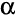# Planned ANOVA-Type Tests

A planned ANOVA-Type Test occurs when appropriate data is selected when pressing(i.e., when conducting Planned Tests Of Statistical Significance).

## Selecting data for conducting ANOVA-Type Tests

The two screenshots below illustrate different examples of selecting data to conduct ANOVA-Type tests. The first of these examples results in a One-Way ANOVA with independent samples and the second in Repeated Measures ANOVA. Refer to ANOVA-Type Tests - Comparing Three or More Groups for details about how Q determines which test to conduct.

## Outputs

### Overall test of significance

The top of the outputs contain the results of the ANOVA-Type Test .

Where a repeated measusures ANOVA is conducted, some 'bonus' tests are added(each differs in their assumptions regarding the violation of the homogeneous variance assumption of ANOVA), and four tests are presented:

### Column comparison details

This contains the details used to compute Column Comparisons: the difference between the means (or proportions) being compared and the Corrected p.

### Homogeneous subsets analysis

The homogeneous subsets are computed by;

• Ordering each of the means (or proportions), from lowest to highest.
• Starting with the lowest mean (or proportion) forming a group that includes this mean (or proportion) and any others that are not significantly different.
• Moving to the next mean (or proportion) that is significantly different (i.e., not in the previous group) and forming a new group that contains all the means (or proportions) that are not different from this mean.
• Etc.